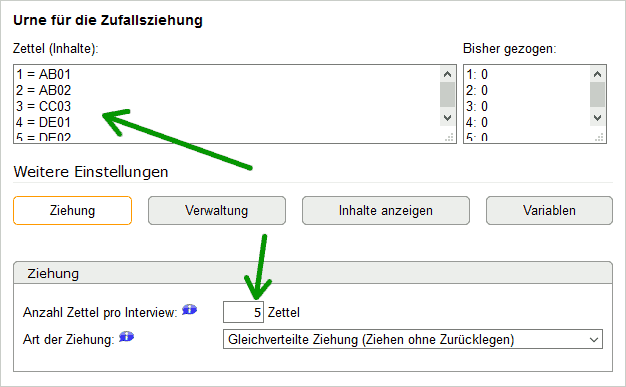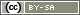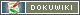# Rotate Questions

In the following it is explained how you can rotate the order of full questions. Therefore you need PHP code. So if you never worked with PHP codes it might be helpful to read the instruction Introduction to PHP.

Tip: If you want to always display certain questions together, follow the instructions Rotate Pages.

Tip: If you only want to rotate the items of a question, please read the chapter Rotation: Items.

There are two ways to rotate questions:

1. For most cases, the simple solution rotation by random generator is more suitable.
2. The more complicated solution to rotation using ''shuffle()'' is described below.

In any case, you create the questions to be rotated in advance in the Question Catalog.

## Rotation by Random Generator

Create a Random Generator in the question catalog. In the random generator write in the Codes(Contents) the question IDs which should rotated be scanned.

```AB01
AB02
CC03
DE01
DE02```

In addition, enter the number of questions to be rotated under More settings in the Draw tab under Number of slips per interview. So in the example above with 5 questions write a 5.### Present Questions

Important! The questions to be presented in random order are not included in the questionnaire!

Instead, when Composing Questionnaire, the random generator is placed in the questionnaire and a PHP code is placed below it. How exactly the PHP code looks depends on whether the questions should appear together on one page or separately on separate pages.

Place the random generator and the PHP code at the place in the questionnaire where you want the questions to be displayed. Regardless of whether you display the questions together or on separate pages, you only need one page for this when Composing Questionnaire.

### Questions together on one Page

At the top of the page place the random generator (in the following example it has the identifier RG01) and the following PHP code below:

```\$questions = valueList('RG01', NULL, 'label');

foreach (\$questions as \$identifier) {
question(\$identifier);
}```

The function `valueList()` retrieves the shuffled list of questions drawn by the random number generator with the identifier “RG01” and stores it in the variable `\$questions`.

The `foreach`-loop then automatically calls the function `question()` for each question identifier, which displays the question. This means that you no longer have to enter the questions separately. You can use the PHP code in exactly the same way and only have to enter the question identifier of your random generator.

### Questions on seperated Pages

To display the questions separately on individual pages, use the command `loopPage()` in the PHP code instead of a `foreach` loop..

```\$questions = valueList('RG01', NULL, 'label');

\$identifier = loopPage(\$questions);
question(\$identifier);```

Thus you have already ensured the rotated sequence of questions. Also, the random generator automatically saves the presentation sequence. The following part of the tutorial explains an alternative solution.

## Rotation by shuffle()

The rotation of questions is in principle also possible without a random generator using the PHP command `shuffle()`. In this case, the presentation order is not saved.

The following example shows how to rotate the order of 5 questions on one page in the questionnaire (for explanation of arrays see Transfer items to another question).

```// Part 1: Create list of questions and mix them
if (!isset(\$questions)) {
// Define list of question IDs
\$questions = [
'AB01',
'AB02',
'CC03',
'DE01',
'DE02'
];
// Mix list randomly
shuffle(\$questions);
// Cache the rotation for possible repetition of the page
registerVariable(\$questions);
}

// Part 2: Present questions
foreach (\$questions as \$identifier) {
question(\$identifier);
}```

The IF construction in part 1 in combination with `isset()` and `registerVariable()` is responsible that the rotation is done only once in the whole interview. This is important if the page is accessed again (e.g. because a question was not answered or because you allow a “back” button) or if the questions should be presented on separate pages.

Note: If you use such PHP codes in different places in the questionnaire, then use a different variable name (e.g. `\$questions1`, `\$questions2`, `\$questions3`) for the list of questions (above: `\$questions`) in each case. Otherwise, the `isset()` ensures that no new question list is defined.

If you want to display the questions separately on separate pages, change the second part as follows.

```// Part 2: Present questions
\$i = loopPage(count(\$questions));
question(\$questions[\$i]);```

## Rotate Questions Together with Other Content

One step further must be taken to rotate additional data (e.g., a picture as a stimulus) along with the questions. So if you always want to show “image1.png” before question 1 and “image2.gif” before question 2. But in principle nothing changes. In the following example, each page shows only one combination of image and question.

Tip: If you want to program less, you can find a simpler solution in the tutorial Rotate Pages.

```if (!isset(\$blocks)) {
// List with question IDs and associated images
\$blocks = [
['AB01', 'image1.png'],
['AB02', 'image2.gif'],
['AB03', 'image3.jpg'],
['AB04', 'image4.png']
];

// Mix list
shuffle(\$blocks);
// Make the lists available on all pages
registerVariable(\$blocks);
}

// Present blocks
\$i = loopPage(count(\$blocks));

// Display image
html('<p><img src="'.\$blocks[\$i].'" alt="Election poster"></p>');

// Display question
question(\$blocks[\$i]);```